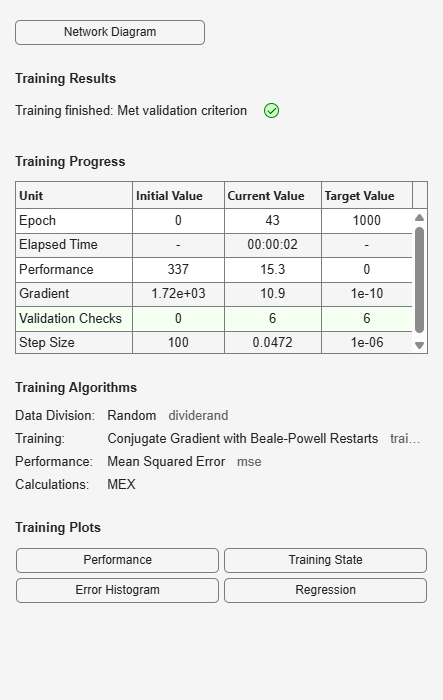# traincgb

Conjugate gradient backpropagation with Powell-Beale restarts

## Syntax

```net.trainFcn = 'traincgb' [net,tr] = train(net,...) ```

## Description

`traincgb` is a network training function that updates weight and bias values according to the conjugate gradient backpropagation with Powell-Beale restarts.

`net.trainFcn = 'traincgb'` sets the network `trainFcn` property.

`[net,tr] = train(net,...)` trains the network with `traincgb`.

Training occurs according to `traincgb` training parameters, shown here with their default values:

 `net.trainParam.epochs` `1000` Maximum number of epochs to train `net.trainParam.show` `25` Epochs between displays (`NaN` for no displays) `net.trainParam.showCommandLine` `false` Generate command-line output `net.trainParam.showWindow` `true` Show training GUI `net.trainParam.goal` `0` Performance goal `net.trainParam.time` `inf` Maximum time to train in seconds `net.trainParam.min_grad` `1e-10` Minimum performance gradient `net.trainParam.max_fail` `6` Maximum validation failures `net.trainParam.searchFcn` `'srchcha'` Name of line search routine to use

Parameters related to line search methods (not all used for all methods):

 `net.trainParam.scal_tol` `20` Divide into `delta` to determine tolerance for linear search. `net.trainParam.alpha` `0.001` Scale factor that determines sufficient reduction in `perf` `net.trainParam.beta` `0.1` Scale factor that determines sufficiently large step size `net.trainParam.delta` `0.01` Initial step size in interval location step `net.trainParam.gama` `0.1` Parameter to avoid small reductions in performance, usually set to `0.1` (see `srch_cha`) `net.trainParam.low_lim` `0.1` Lower limit on change in step size `net.trainParam.up_lim` ` 0.5` Upper limit on change in step size `net.trainParam.maxstep` `100` Maximum step length `net.trainParam.minstep` `1.0e-6` Minimum step length `net.trainParam.bmax` `26` Maximum step size

## Network Use

You can create a standard network that uses `traincgb` with `feedforwardnet` or `cascadeforwardnet`.

To prepare a custom network to be trained with `traincgb`,

1. Set `net.trainFcn` to `'traincgb'`. This sets `net.trainParam` to `traincgb`’s default parameters.

2. Set `net.trainParam` properties to desired values.

In either case, calling `train` with the resulting network trains the network with `traincgb`.

## Examples

collapse all

This example shows how to train a neural network using the `traincgb` train function.

Here a neural network is trained to predict body fat percentages.

```[x, t] = bodyfat_dataset; net = feedforwardnet(10, 'traincgb'); net = train(net, x, t);````y = net(x);`

collapse all

### Powell-Beale Algorithm

For all conjugate gradient algorithms, the search direction is periodically reset to the negative of the gradient. The standard reset point occurs when the number of iterations is equal to the number of network parameters (weights and biases), but there are other reset methods that can improve the efficiency of training. One such reset method was proposed by Powell [Powe77], based on an earlier version proposed by Beale [Beal72]. This technique restarts if there is very little orthogonality left between the current gradient and the previous gradient. This is tested with the following inequality:

`$|{g}_{k-1}^{T}{g}_{k}|\ge 0.2{‖{g}_{k}‖}^{2}$`

If this condition is satisfied, the search direction is reset to the negative of the gradient.

The `traincgb` routine has somewhat better performance than `traincgp` for some problems, although performance on any given problem is difficult to predict. The storage requirements for the Powell-Beale algorithm (six vectors) are slightly larger than for Polak-Ribiére (four vectors).

## Algorithms

`traincgb` can train any network as long as its weight, net input, and transfer functions have derivative functions.

Backpropagation is used to calculate derivatives of performance `perf` with respect to the weight and bias variables `X`. Each variable is adjusted according to the following:

```X = X + a*dX; ```

where `dX` is the search direction. The parameter `a` is selected to minimize the performance along the search direction. The line search function `searchFcn` is used to locate the minimum point. The first search direction is the negative of the gradient of performance. In succeeding iterations the search direction is computed from the new gradient and the previous search direction according to the formula

```dX = -gX + dX_old*Z; ```

where `gX` is the gradient. The parameter `Z` can be computed in several different ways. The Powell-Beale variation of conjugate gradient is distinguished by two features. First, the algorithm uses a test to determine when to reset the search direction to the negative of the gradient. Second, the search direction is computed from the negative gradient, the previous search direction, and the last search direction before the previous reset. See Powell, Mathematical Programming, Vol. 12, 1977, pp. 241 to 254, for a more detailed discussion of the algorithm.

Training stops when any of these conditions occurs:

• The maximum number of `epochs` (repetitions) is reached.

• The maximum amount of `time` is exceeded.

• Performance is minimized to the `goal`.

• The performance gradient falls below `min_grad`.

• Validation performance (validation error) has increased more than `max_fail` times since the last time it decreased (when using validation).

## References

Powell, M.J.D., “Restart procedures for the conjugate gradient method,” Mathematical Programming, Vol. 12, 1977, pp. 241–254

## Version History

Introduced before R2006a